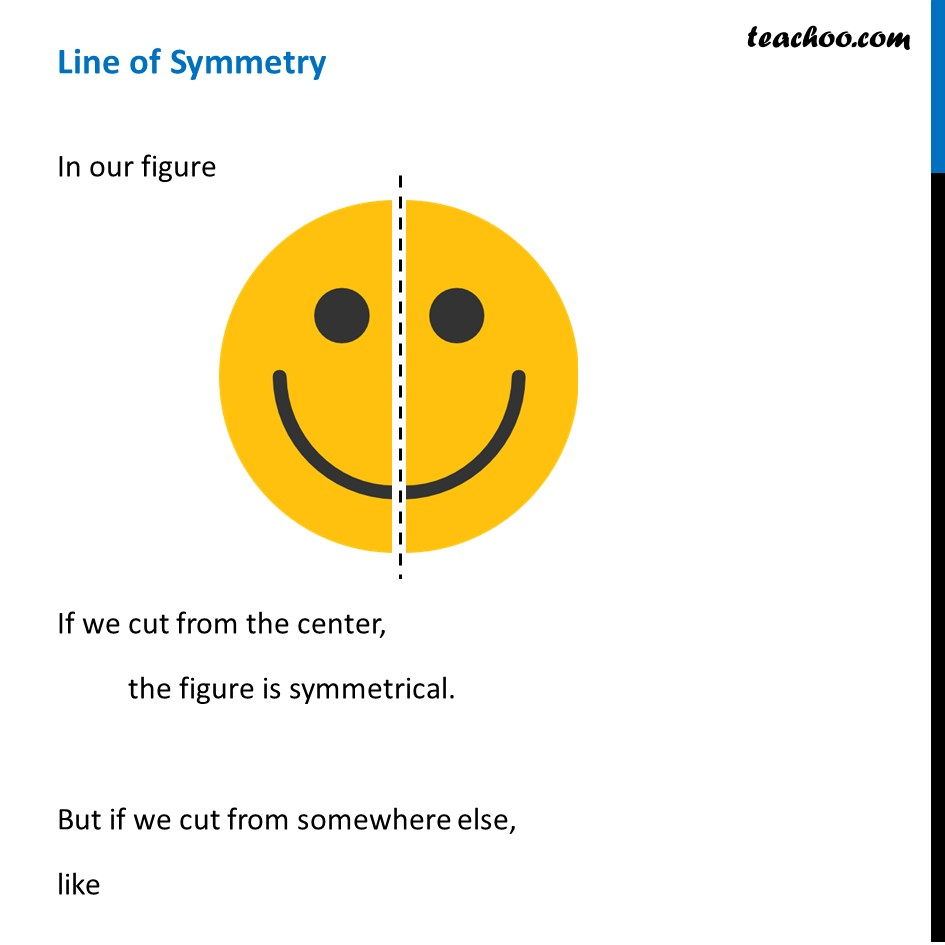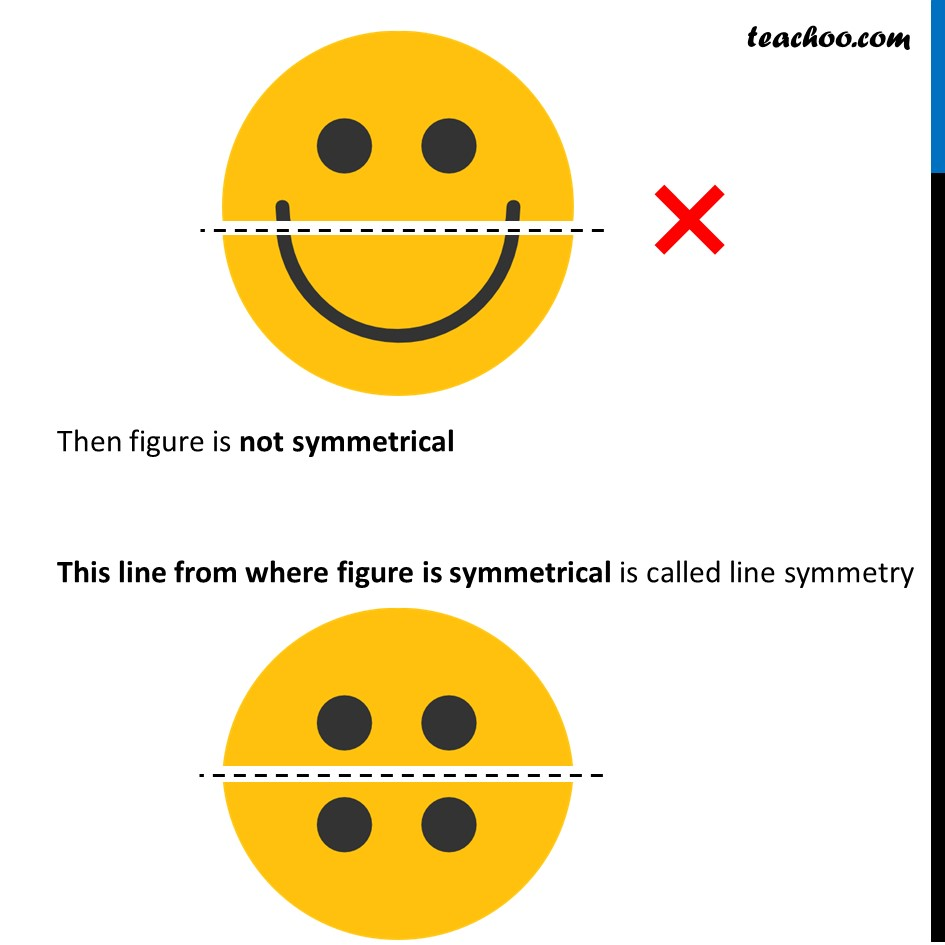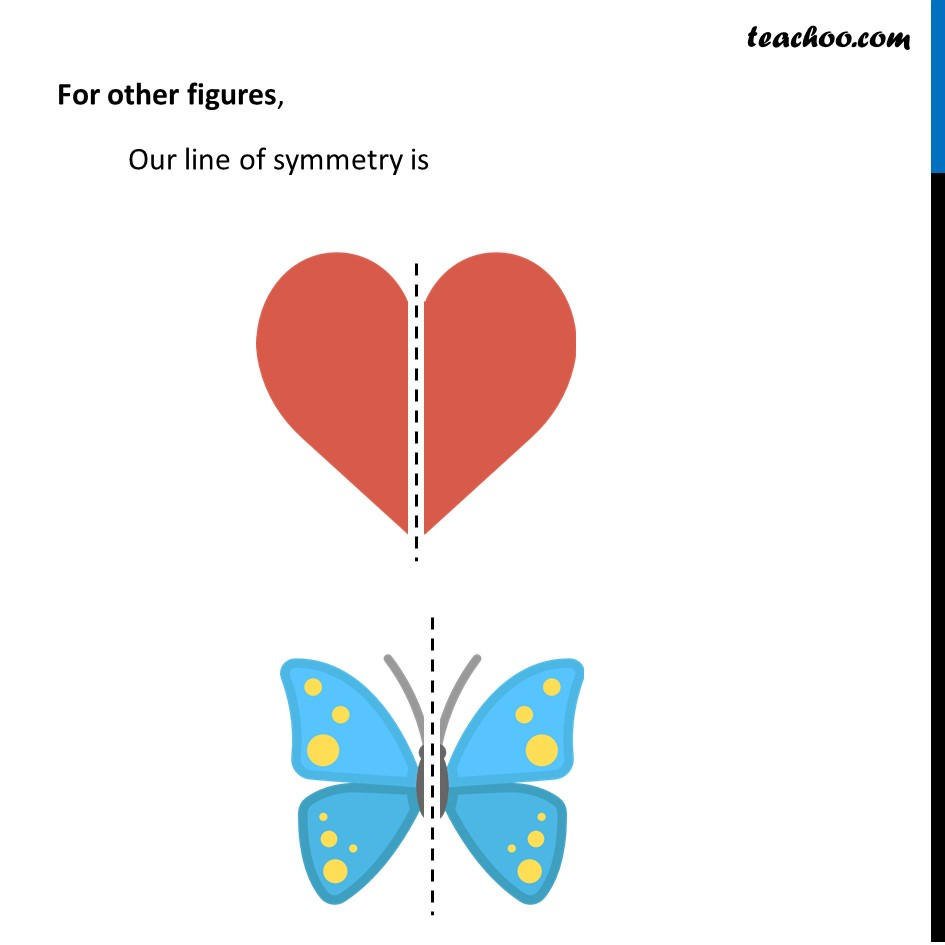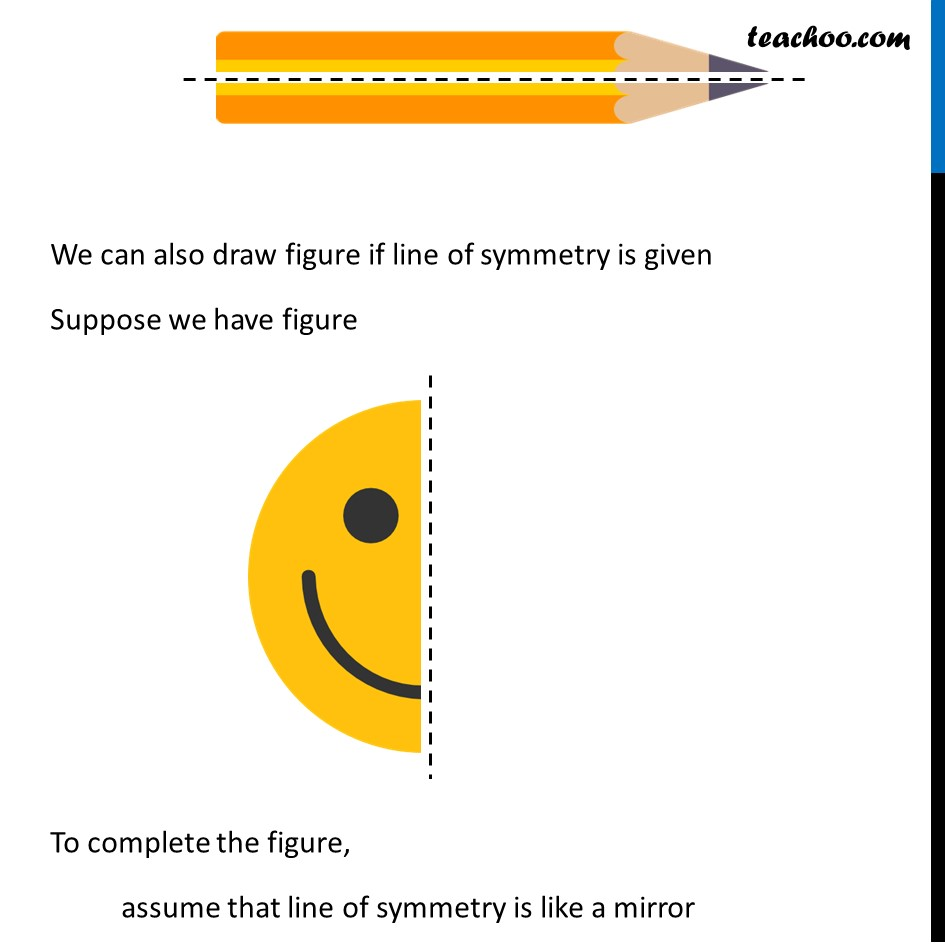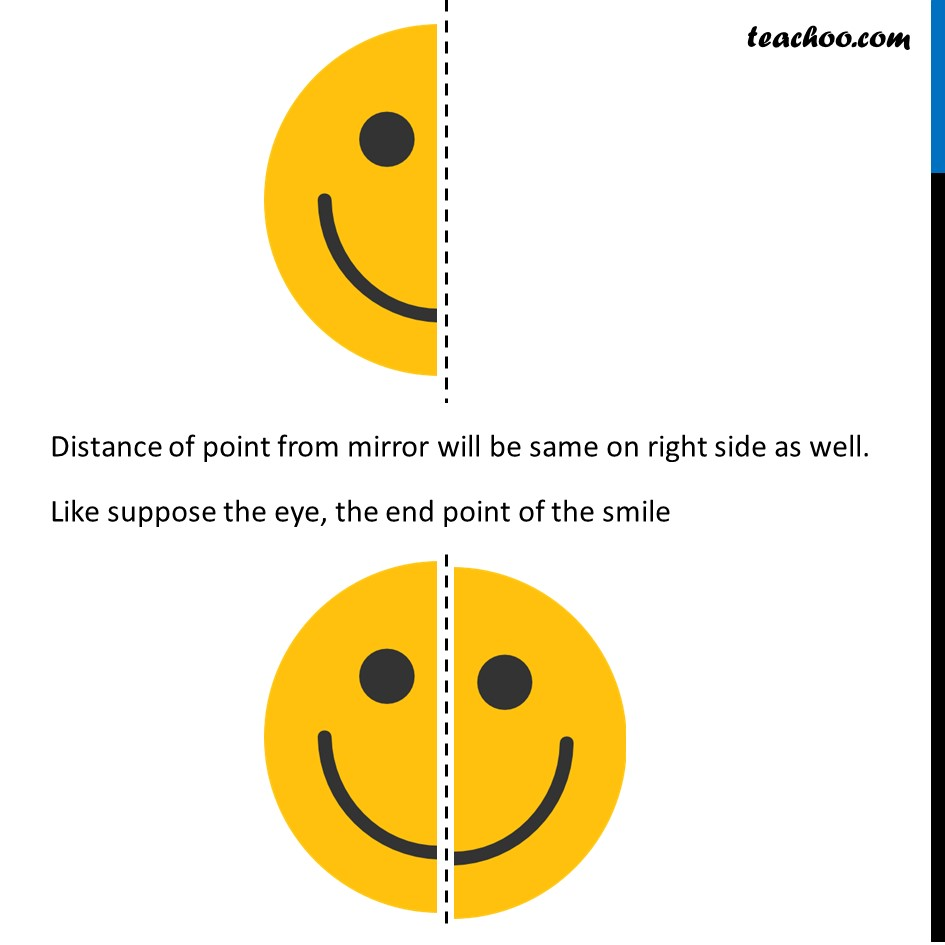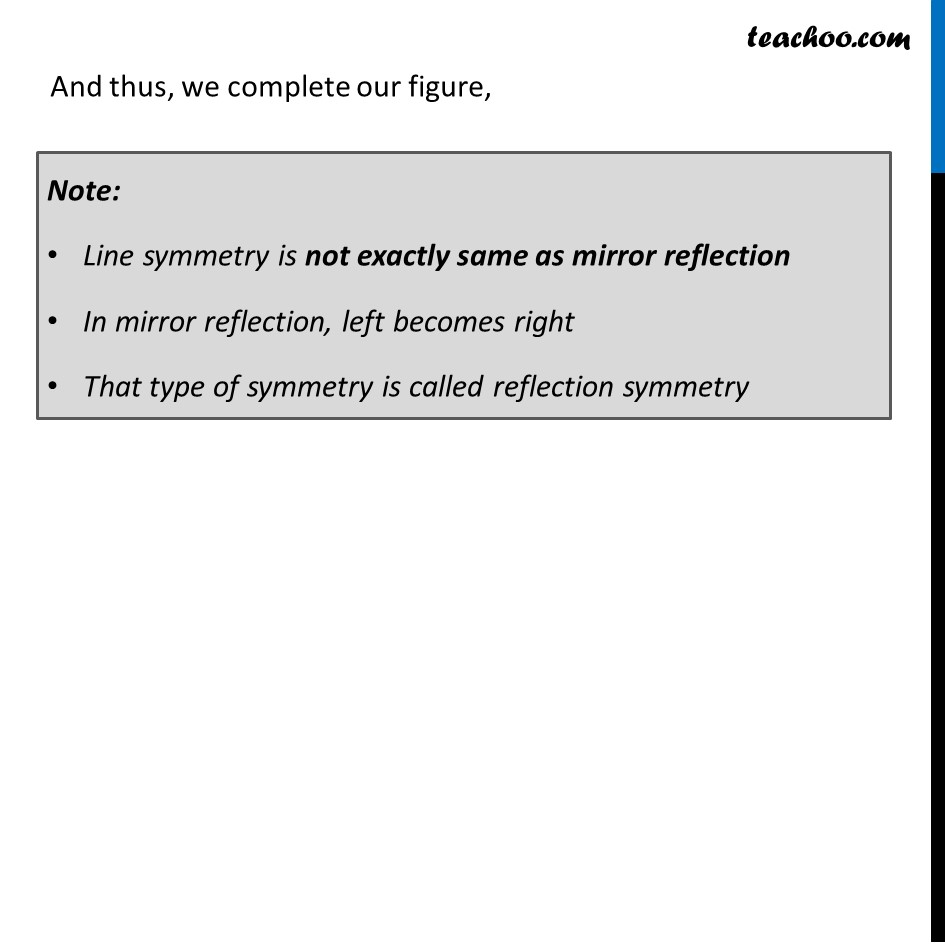1. Chapter 13 Class 6 Symmetry
2. Concept wise
3. Line of symmetry

Transcript

Line of SymmetryIn our figure If we cut from the center, the figure is symmetrical. But if we cut from somewhere else, like Then figure is not symmetrical This line from where figure is symmetrical is called line symmetry For other figures, Our line of symmetry is We can also draw figure if line of symmetry is given Suppose we have figure To complete the figure, assume that line of symmetry is like a mirror Distance of point from mirror will be same on right side as well. Like suppose the eye, the end point of the smile And thus, we complete our figure, Note: Line symmetry is not exactly same as mirror reflection In mirror reflection, left becomes right That type of symmetry is called reflection symmetry

Line of symmetry

About the AuthorDavneet Singh
Davneet Singh is a graduate from Indian Institute of Technology, Kanpur. He has been teaching from the past 10 years. He provides courses for Maths and Science at Teachoo.